085-1263404

### My Journal

All things Mathematical
15 Nov 2018

#### Question 1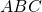is a triangle in which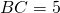cm.,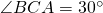, and. Forces of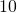,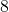,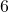lb. wt. act along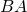,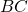,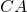, respectively. If their resultant acts along the straight line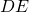, cuttingin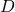, calculate the length of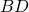and the size of the angle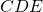.

#### Question 2

Show that the centre of gravity of a triangular lamina is the same as that of three equal masses situated at the vertices of the triangle.

A lamina is in the shape of a trapeziumin which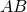and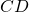are parallel.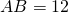,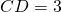,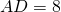and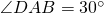. Find the perpendicular distance of the centre of gravity of the lamina (i) from, and (ii) from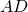.

#### Question 3

Explain the terms “limiting friction”, “angle of friction”.

A horizontal force of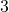lb. wt. would just keep a block of mass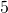lb. from sliding down a rough plane which is inclined to the horizontal at an angle of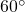. Calculate the coefficient of friction between the block and the plane.

Find the direction and magnitude of the least force that would keep the block from sliding down the plane.

#### Question 4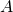,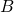,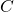are three ships at sea. To an observer on,appears to be travelling South-East atknots. To an observer on,appears to be travelling due North atknots. Find the velocity ofrelative toin magnitude and direction.

#### Question 5

A car travelling on a level road was uniformly accelerated for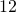seconds and was then uniformly retarded for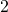seconds. In that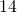seconds it travelled fromto, a distance of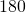yards. Its velocity atwas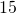m.p.h. and its velocity atwas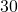m.p.h. Find the uniform acceleration and the uniform retardation, in feet per sec..

Calculate the horse-power at which the car was working when its velocity was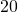m.p.h., given that the car weighed one ton and that the frictional resistances to motion were equivalent to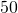lb. wt.

#### Question 6

A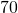gm. mass is projected vertically upwards with an initial velocity of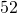ft. per sec. and half a second later a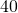gm. mass is projected vertically upwards from the same point with an initial velocity of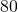ft. per sec. Calculate the height at which the masses will collide.

If the masses coalesce on colliding, find the greatest height which the combined mass will reach and the velocity it will have on returning to the point of projection.

#### Question 7

If a body is describing a circle of radius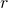with constant angular velocity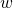, show that its acceleration is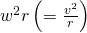directed towards the centre of the circle.

A particle of mass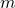, suspended from a fixed point by a string of length, is describing a horizontal circle with uniform angular velocity. Express in terms of,m(i) the tension in the string, (ii) the cosine of the angle which the string makes with the vertical.

Show that the time taken by the particle in making one revolution is less than the time of oscillation of a simple pendulum of length.

#### Question 8

Define simple harmonic motion.

A particle is moving along a straight line. At time(secs.) its distance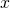(cms.) from its mean position is given by the formula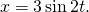Show that the motion is simple harmonic and find the periodic time.

Calculate the velocity of the particle (i) when it is in its mean position, (ii) half a second later.

#### Question 9

There arefeet of water on one side of a lock-gate andfeet of water on the other. The gate is rectangular and isfeet wide. Calculate the resultant thrust of the water on the date, in tons.

By how much should the level of the water on the deeper side be lowered so that the resultant thrust would be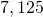lb. less?

[A cubic foot of water weighs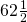lb.]

##### Citation:

State Examinations Commission (2018). State Examination Commission. Accessed at: https://www.examinations.ie/?l=en&mc=au&sc=ru

Malone, D and Murray, H. (2016). Archive of Maths State Exams Papers. Accessed at: http://archive.maths.nuim.ie/staff/dmalone/StateExamPapers/

##### Licence:

“Contains Irish Public Sector Information licensed under a Creative Commons Attribution 4.0 International (CC BY 4.0) licence”.

The EU Directive 2003/98/EC on the re-use of public sector information, its amendment EU Directive 2013/37/EC, its transposed Irish Statutory Instruments S.I. No. 279/2005, S.I No. 103/2008, and S.I. No. 525/2015, and related Circulars issued by the Department of Finance (Circular 32/05), and Department of Public Expenditure and Reform (Circular 16/15 and Circular 12/16).

Note. Circular 12/2016: Licence for Re-Use of Public Sector Information adopts CC-BY as the standard PSI licence, and notes that the open standard licence identified in this Circular supersedes PSI General Licence No: 2005/08/01.

Links:

https://circulars.gov.ie/pdf/circular/per/2016/12.pdf

https://creativecommons.org/licenses/by/4.0/legalcod

This site uses Akismet to reduce spam. Learn how your comment data is processed.